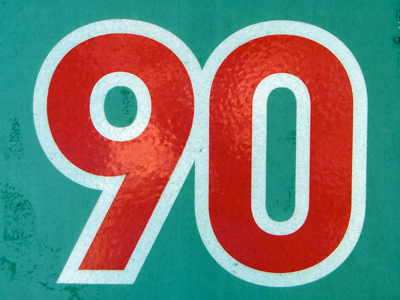10 x 9 = 90.

# 10 Times Table

Learning the times tables is a vital tool when multiplying numbers. By the end of Year 2 children are often fluent in their times tables up to 10. When they enter into KS2 in Year 3 they will also be taught some higher times tables in their Maths and Numeracy lessons. By the end of KS2 they will be familiar with all of the times tables from one to twelve. This quiz will test their knowledge of the 10 times table.

Multiplying numbers by 10 is what the 10 times table is all about! Along with the times tables for the low numbers, like 2, 3 and 4, learning the 10 times table is really very easy - to multiply a number by 10 just add a 0 to the end!

Learning your times tables is easier with practise. Practise your 10 times table by playing this quiz.

1.
7 x 10 =
70
75
80
85
70 mph is the speed limit on motorways and dual carriageways
2.
5 x 10 =
10
15
40
50
5 is half of 10 so 50 is half of 100
3.
10 x 11 =
100
105
110
111
4.
10 x 3 =
13
30
31
33
To multiply any number by 10 simply add a 0 to its end
5.
10 x 12 =
100
110
120
130
6.
10 x 9 =
90
100
110
120
7.
10 x 2 =
20
22
25
27
8.
8 x 10 =
80
81
87
88
9.
6 x 10 =
30
40
50
60
There are 60 seconds in a minute and 60 minutes in an hour
10.
10 x 4 =
10
27
40
44

Author:  Amanda Swift